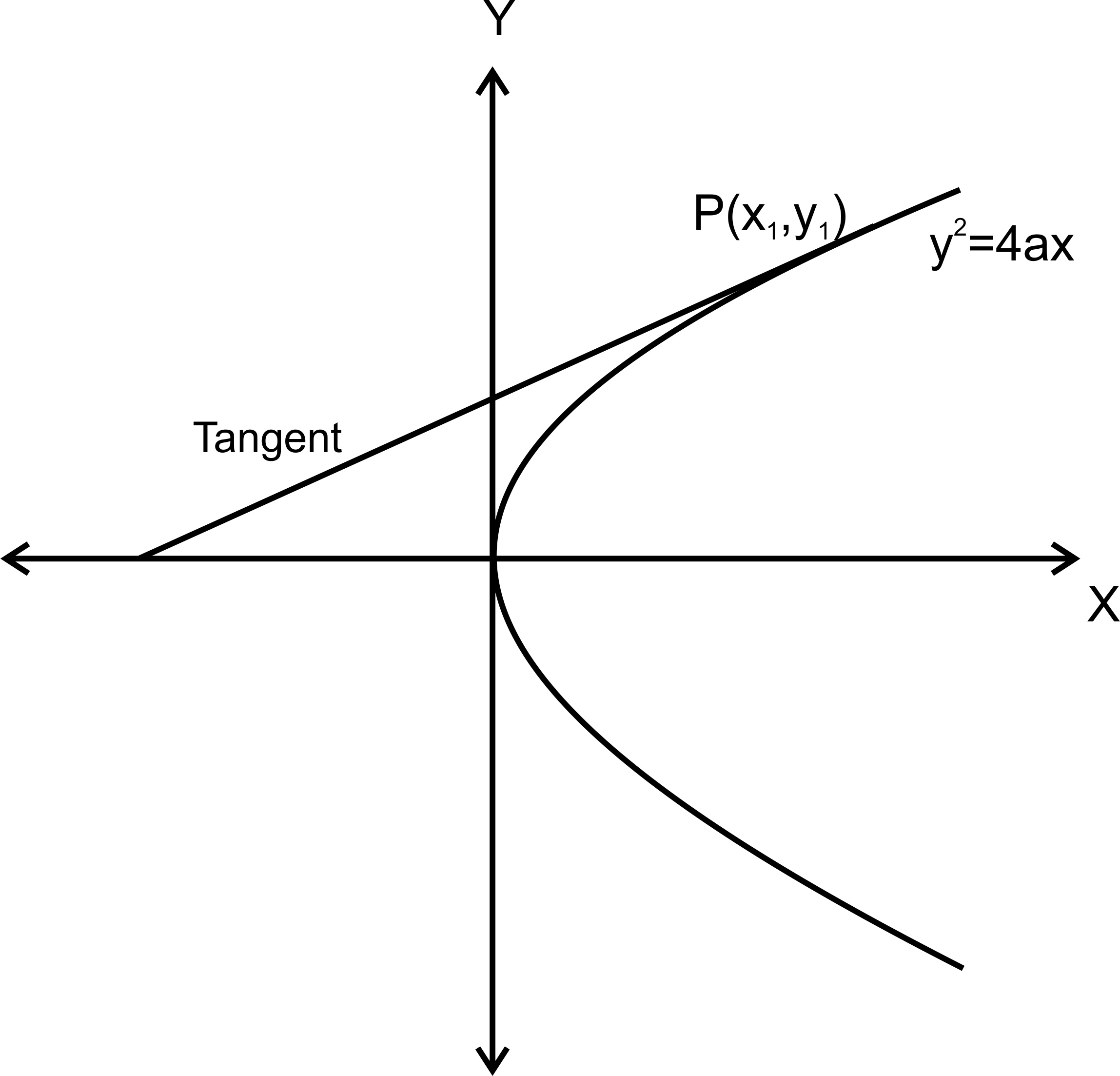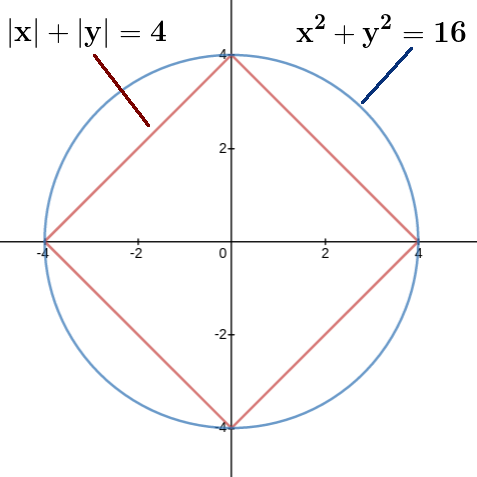Filter By

7/12

225

## Crack CUET with india's "Best Teachers"

• HD Video Lectures
• Unlimited Mock Tests
• Faculty Support#### If one end of a focal chord AB of the parabolais atthen the equation of the tangent to it at B is : Option: 1Option: 2Option: 3Option: 4Length of the Latus rectum and parametric form -

Parametric Equation:

From the equation of the parabola, we can write-

Tangents of Parabola in Point Form -

Tangents of Parabola in  Point Form-Correct Option 3

Option: 3

## Crack NEET with "AI Coach"

• HD Video Lectures
• Unlimited Mock Tests
• Faculty Support#### If  for  then Option: 1Option: 2Option: 3Option: 4Use

Correct Option (3)

#### Ifbe a complex number satisfyingthencannot be :  Option: 1Option: 2Option: 3Option: 4Complex number -

A complex number is the sum of a real number and an imaginary number. A complex number is expressed in standard form as a + bi where a is the real part and b is the imaginary part. For example, 5 + 2i is a complex number. So, too, is 3 + 4i√3.We write the complex number by C or z = a + ib, a and b are real number (a, b ∈ R).

• a is real part of the complex number and denoted by Re(z),

• b is the imaginary part of the complex number and denoted by Im(z),

E.g :    z = 2 + 3i is a complex number.

With Re(z) = 2 and Im(z) = 3

-

Area of triangle, circle (formula) -

Equation of Circle:

The equation of the circle whose center is at the pointand have radius r is given byIf the center is origin then,, hence equation reduces to |z| = r

Interior of the circle is represented byThe exterior is represented byHere z can be represented as x + iy andis represented by-

z = x + iy

|x| + |y| = 4Minimum value of |z| =Maximum value of |z| = 4So |z| can't beCorrect Option (1)

## Crack JEE Main with "AI Coach"

• HD Video Lectures
• Unlimited Mock Tests
• Faculty Support#### The length of the minor axis (along y-axis) of an ellipse in the standard form isIf this ellipse touches the line,then its eccentricity is :  Option: 1Option: 2Option: 3Option: 4What is Ellipse? -

Ellipse

Standard Equation of Ellipse:

The standard form of the equation of an ellipse with center (0, 0) and major axis on the x-axis is1. a > b

2.  the length of the major axis is 2a

3.  the coordinates of the vertices are (±a, 0)

4.  the length of the minor axis is 2b

5.  the coordinates of the co-vertices are (0, ±b)

-

Equation of Tangent of Ellipse in Parametric Form and Slope Form -

Slope Form:-